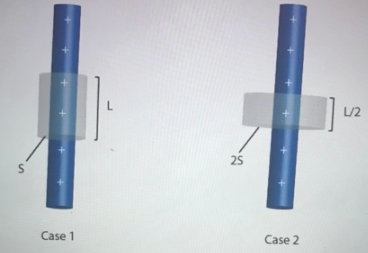# Problem: An infinitely long charged rod has uniform charge density of lambda, and passes through a cylinder (gray). The cylinder in case 2 has twice the radius and half the length compared to the cylinder in case 1. Compare the magnitude of the flux, Phi, through the surface of the cylinder in both cases. a. Phi1 = 2Phi2b. Phi2 Phi1 = Phi2 c. Phi1 = 1/2 Phi2 d. None of these

###### FREE Expert Solution

The total flux is given by:

$\overline{){\mathbf{\varphi }}{\mathbf{=}}\frac{\mathbf{\lambda }\mathbf{L}}{{\mathbf{\epsilon }}_{\mathbf{0}}}}$, where λ is charge density, L is the length of the rod, and ε0 is the permittivity of free space.

The flux for the first rod is given by:

${{\mathbf{\varphi }}}_{{\mathbf{1}}}{\mathbf{=}}\frac{\mathbf{\lambda L}}{{\mathbf{\epsilon }}_{\mathbf{0}}}$

98% (479 ratings)###### Problem Details

An infinitely long charged rod has uniform charge density of lambda, and passes through a cylinder (gray). The cylinder in case 2 has twice the radius and half the length compared to the cylinder in case 1. Compare the magnitude of the flux, Phi, through the surface of the cylinder in both cases.a. Phi1 = 2Phi2

b. Phi2 Phi1 = Phi2

c. Phi1 = 1/2 Phi2

d. None of these